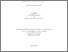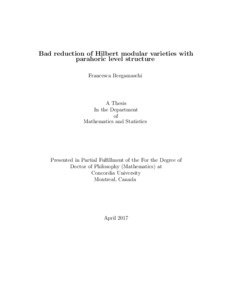Title:

# Bad reduction of Hilbert modular varieties with parahoric level structure

Bergamaschi, Francesca (2017) Bad reduction of Hilbert modular varieties with parahoric level structure. PhD thesis, Concordia University.Preview Text (application/pdf) Bergamaschi_PhD_S2017.pdf - Accepted Version Available under License Spectrum Terms of Access. 924kB

## Abstract

Abelian varieties can be thought of as a higher dimensional analogue to elliptic curves. Over fields, they are defined as complete algebraic varieties with a compatible group structure. One of the most fertile fields in arithmetic geometry is concerned with the study of abelian varieties in prime characteristic. The fundamental reason why this area has become so central is that the many interesting phenomena arising in positive characteristic provide us with very powerful geometric tools. For instance, several results in characteristic zero can be derived studying the reduction to positive characteristic.

A very fruitful approach for describing such phenomena is to look at several abelian varieties at once. Roughly speaking, the abelian varieties of a given dimension are seen as points of a space, namely a \emph{moduli space}. In general, in order for these spaces to have \emph{nice} geometric properties, we will expect the abelian varieties to have additional structure, such as, for example, some data on the $N$-torsion (kernel of the multiplication by $N$ map, for some positive integer $N$), called a structure of level $N$. The fundamental example in this context is that of modular curves that is, spaces whose points parametrize (isomorphism classes of) elliptic curves with some level $N$ structure. The work of the author focuses on a generalization of modular curves, that is, Hilbert modular varieties, parametrizing abelian varieties with polarization, $N$-level structure and real multiplication, that is, an action by a suitable finite field extension of $\Q$.

The most immediate questions arising from the study of such spaces are purely of geometric nature. The geometry of Hilbert modular varieties is well understood in every characteristic $p$, as long as $p$ does not divide the level structure. When $p$ divides the level structure however, things get complicated. In this thesis we provide a description of Hilbert modular varieties in the case when $p$ divides the level $N$ (case of \emph{parahoric} level). In particular, we obtain an understanding of these spaces by giving a full description of the deformation of abelian varieties and by defining suitable \emph{stratifications} depending on the $p$-torsion of abelian varieties.

Divisions: Concordia University > Faculty of Arts and Science > Mathematics and Statistics Thesis (PhD) Bergamaschi, Francesca Concordia University Ph. D. Mathematics April 2017 Iovita, Adrian and Edixhoven, Bas and Andreatta, Fabrizio Mathematics, Arithmetic Geometry, Hilbert Modular Varieties 982542 FRANCESCA BERGAMASCHI 01 Jun 2017 12:32 18 Jan 2018 17:55
All items in Spectrum are protected by copyright, with all rights reserved. The use of items is governed by Spectrum's terms of access.

Repository Staff Only: item control pageResearch related to the current document (at the CORE website)
Back to top